# Turbofan calculator for public use

Turbofan calculator. The formulae correspond to P.G.Hill and C.R. Peterson, "Mechanics and Thermodynamics of Propulsion", Addison-Wesley, 1970. The sketch explains the notation.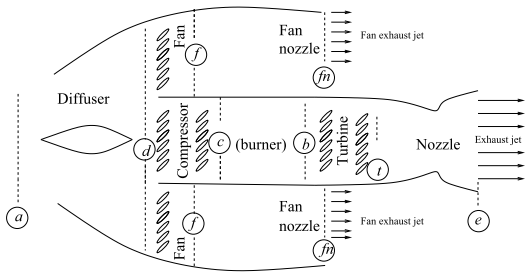Diffuser input:
Specific heat ratio γ= (for air 1.4)
Flight Mach number M =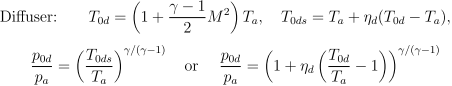Diffuser output:
Stagnation temperature/ambient temperature T0d/Ta=
Stagnation pressure/ambient pressure p0d/pa=

Compressor input:
Compressor pressure ratio prc=
Compressor specific heat ratio γc=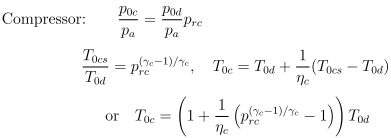Compressor output:
Stagnation temperature/ambient temperature T0c/Taa =
Stagnation pressure/ambient pressure p0c/pa=

Fan input:
Fan pressure ratio prf=
Fan specific heat ratio γf=
Gas constant (fan nozzle) Rf= in J/(Kg K). (For dry air it is around 287.)
Ambient temperature Ta= in grad K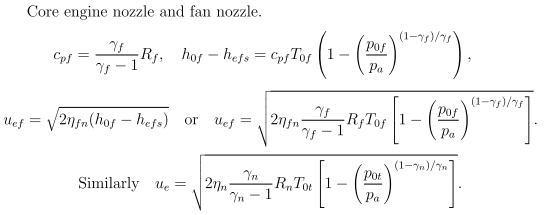Fan output:
Stagnation temperature/ambient temperature T0f/Ta=
Stagnation pressure/ambient pressure p0f/pa=
Fan nozzle exhaust velocity uef=m/s

Burner input:
Burner pressure ratio rb=
Stagnation temperature T0b= in grad K
Effective heat of reaction ηb QR= in kJ/kg (for typical aviation kerosene QR=45000 kJ/kg)
Specific heat ratio of the combustion products cp= in J/(kg K) (for dry air it is around 1005)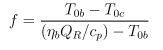Burner output:
Fuel-to-air ratio f=
Stagnation temperature/ambient temperature T0b/Ta=
Stagnation pressure/ambient pressure p_0b/p_a=

Turbine input:
Specific heat ratio (turbine) γt=
Bypass ratio β=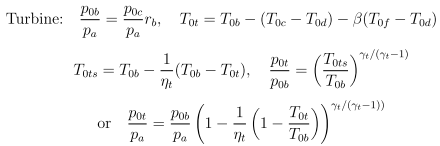Turbine output:
Stagnation temperature/ambient temperature T0t/Ta=
Stagnation pressure/ambient pressure p0t/pa=

Nozzle input:
Specific heat ratio (nozzle) γn=
Gas constant (nozzle) Rn= in J/(kg K)
Gas constant (air) R= in J/(kg K)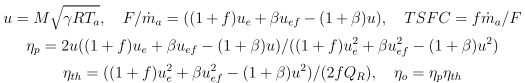Nozzle output:
Stagnation pressure/ambient pressure p0e/pa=
Exhaust stagnation temperature T0e= in grad K
Exhaust velocity ue= m/s
Flight velocity u= m/s
Specific Thrust = kN/(kg/s)
TSFC= (kg/s)/kN
Propulsion efficiency ηp=
Thermal efficiency ηthb x
Overall efficiency ηob x